xGeopotential heightEncyclopedia
Geopotential height is a vertical coordinate referenced to Earth's mean sea level — an adjustment to geometric height (elevation
Elevation
The elevation of a geographic location is its height above a fixed reference point, most commonly a reference geoid, a mathematical model of the Earth's sea level as an equipotential gravitational surface ....

above mean sea level) using the variation of gravity with latitude
Latitude
In geography, the latitude of a location on the Earth is the angular distance of that location south or north of the Equator. The latitude is an angle, and is usually measured in degrees . The equator has a latitude of 0°, the North pole has a latitude of 90° north , and the South pole has a...

and elevation. Thus it can be considered a "gravity-adjusted height". One usually speaks of the geopotential height of a certain pressure level, which would correspond to the geopotential height necessary to reach the given pressure
Atmospheric pressure
Atmospheric pressure is the force per unit area exerted into a surface by the weight of air above that surface in the atmosphere of Earth . In most circumstances atmospheric pressure is closely approximated by the hydrostatic pressure caused by the weight of air above the measurement point...

.

At an elevation of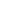, the geopotential is defined as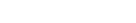where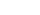is the acceleration due to gravity,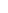is latitude, andis the geometric elevation.
Thus, it is the gravitational potential energy per unit mass at that level. The geopotential height is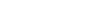where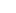is the standard gravity at mean sea level.

Geophysical scientists
Geophysics
Geophysics is the physics of the Earth and its environment in space; also the study of the Earth using quantitative physical methods. The term geophysics sometimes refers only to the geological applications: Earth's shape; its gravitational and magnetic fields; its internal structure and...

often use geopotential height rather than geometric height, because doing so in many cases makes analytical calculations more convenient. For example, the primitive equations
Primitive equations
The primitive equations are a set of nonlinear differential equations that are used to approximate global atmospheric flow and are used in most atmospheric models...

which weather forecast models
Numerical weather prediction
Numerical weather prediction uses mathematical models of the atmosphere and oceans to predict the weather based on current weather conditions. Though first attempted in the 1920s, it was not until the advent of computer simulation in the 1950s that numerical weather predictions produced realistic...

solve are more easily expressed in terms of geopotential than geometric height. Using the former eliminates centrifugal force and air density (which is very difficult to measure) in the equations.

A plot of geopotential height for a single pressure level shows the troughs and ridges, Highs and Lows, which are typically seen on upper air charts. The geopotential thickness between pressure levels — difference of the 850 hPa
Pascal (unit)
The pascal is the SI derived unit of pressure, internal pressure, stress, Young's modulus and tensile strength, named after the French mathematician, physicist, inventor, writer, and philosopher Blaise Pascal. It is a measure of force per unit area, defined as one newton per square metre...

and 1000 hPa geopotential heights for example — is proportional to mean virtual temperature
Virtual temperature
In atmospheric thermodynamics, the virtual temperature T_v of a moist air parcel is the temperature at which a theoretical dry air parcel would have a total pressure and density equal to the moist parcel of air.-Description:...

in that layer. Geopotential height contours can be used to calculate the geostrophic wind
Geostrophic wind
The geostrophic wind is the theoretical wind that would result from an exact balance between the Coriolis effect and the pressure gradient force. This condition is called geostrophic balance. The geostrophic wind is directed parallel to isobars . This balance seldom holds exactly in nature...

, which is faster where the contours are more closely spaced and tangential to the geopotential height contours.